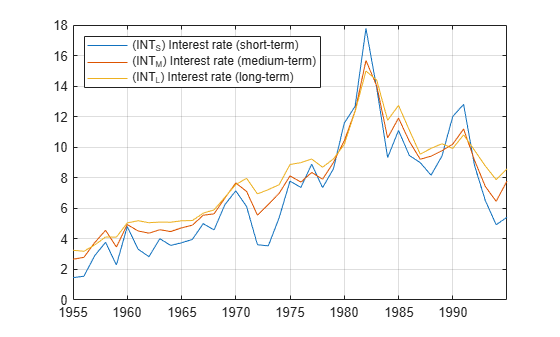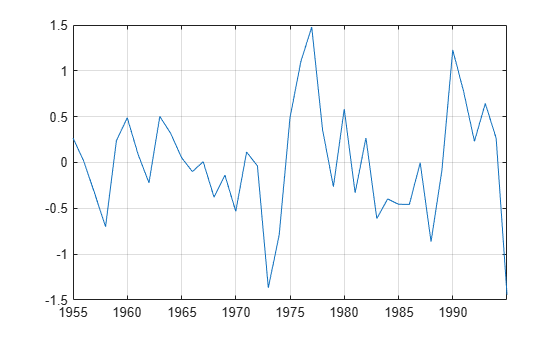# egcitest

Engle-Granger cointegration test

## Syntax

```[h,pValue,stat,cValue,reg1,reg2] = egcitest(Y) [h,pValue,stat,cValue,reg1,reg2] = egcitest(Y,Name,Value) ```

## Description

Engle-Granger tests assess the null hypothesis of no cointegration among the time series in `Y`. The test regresses `Y(:,1)` on `Y(:,2:end)`, then tests the residuals for a unit root.

```[h,pValue,stat,cValue,reg1,reg2] = egcitest(Y)``` performs the Engle-Granger test on a data matrix `Y`.

```[h,pValue,stat,cValue,reg1,reg2] = egcitest(Y,Name,Value)``` performs the Engle-Granger test on a data matrix `Y` with additional options specified by one or more `Name,Value` pair arguments.

## Input Arguments

 `Y` numObs-by-numDims matrix representing numObs observations of a numDims-dimensional time series y(t), with the last observation the most recent. Y cannot have more than 12 columns. Observations containing `NaN` values are removed.

### Name-Value Arguments

Specify optional comma-separated pairs of `Name,Value` arguments. `Name` is the argument name and `Value` is the corresponding value. `Name` must appear inside quotes. You can specify several name and value pair arguments in any order as `Name1,Value1,...,NameN,ValueN`.

 `creg` Character vector, such as `'nc'`, or cell vector of character vectors indicating the form of the cointegrating regression, where y1 = `Y(:,1)` is regressed on Y2 = `Y(:,2:end)` and optional deterministic terms in X:y1 = Xa + Y2b + εValues are: `'nc'`—no constant or trend in X`'c'`—constant but no trend in X`'ct'` —constant and linear trend in X`'ctt'` —constant, linear trend, and quadratic trend in X Default: `'c'` `cvec` Vector or cell vector of vectors containing coefficients [a;b] to be held fixed in the cointegrating regression. The length of a is 0, 1, 2 or 3, depending on `creg`, with coefficient order: constant, linear trend, quadratic trend. The length of b is numDims − 1. It is assumed that the coefficient of y1 = `Y(:,1)` has been normalized to 1. `NaN` values indicate coefficients to be estimated. If `cvec` is completely specified (no `NaN` values), no cointegrating regression is performed. Default: Completely unspecified cointegrating vector (all NaN values). `rreg` Character vector, such as `'ADF'`, or cell vector of character vectors indicating the form of the residual regression. Values are: `'ADF'` — augmented Dickey-Fuller test of residuals from the cointegrating regression`'PP'` — Phillips-Perron test Test statistics are computed by calling `adftest` and `pptest` with the model parameter set to `'AR'`, assuming data have been demeaned or detrended, as necessary, in the cointegrating regression. Default: `'ADF'` `lags` Scalar or vector of nonnegative integers indicating the number of lags used in the residual regression. The meaning of the parameter depends on the value of `rreg` (see the documentation for the `lags` parameter in `adftest` and `pptest`). Default: `0` `test` Character vector, such as `'t1'`, or cell vector of character vectors indicating the type of test statistic computed from the residual regression. Values are: `'t1'` — a “τ test”`'t2'` — a “z test” The meaning of the parameter depends on the value of `rreg` (see the documentation for the test parameter in `adftest` and `pptest`). Default: `t1` `alpha` Scalar or vector of nominal significance levels for the tests. Values must be between 0.001 and 0.999. Default: 0.05

Single-element parameter values are expanded to the length of any vector value (the number of tests). Vector values must have equal length. If any value is a row vector, all outputs are row vectors.

## Output Arguments

`h`

Vector of Boolean decisions for the tests, with length equal to the number of tests. Values of `h` equal to `1` (`true`) indicate rejection of the null in favor of the alternative of cointegration. Values of `h` equal to `0` (`false`) indicate a failure to reject the null.

`pValue`

Vector of p-values of the test statistics, with length equal to the number of tests. p-values are left-tail probabilities.

`stat`

Vector of test statistics, with length equal to the number of tests. The statistic depends on the `rreg` and `test` values (see the documentation for `adftest` and `pptest`).

`cValue`

Vector of critical values for the tests, with length equal to the number of tests. Values are for left-tail probabilities. Since residuals are estimated rather than observed, critical values are different from those used in `adftest` or `pptest` (unless the cointegrating vector is completely specified by `cvec`). `egcitest` loads tables of critical values from the file `Data_EGCITest.mat`, then linearly interpolates test values from the tables. Critical values in the tables were computed using methods described in .

`reg1`

Structure of regression statistics from the cointegrating regression.

`reg2`

Structure of regression statistics from the residual regression.

The number of records in `reg1` and `reg2` equals the number of tests. Each record has the following fields:

 `num` Length of the regression response y, with `NaN`s removed `size` Effective sample size, adjusted for lags, difference* `names` Regression coefficient names `coeff` Estimated coefficient values `se` Estimated coefficient standard errors `Cov` Estimated coefficient covariance matrix `tStats` t statistics of coefficients and p-values `FStat` F statistic and p-value `yMu` Mean of y, adjusted for lags, difference* `ySigma` Standard deviation of y, adjusted for lags, difference* `yHat` Fitted values of y, adjusted for lags, difference* `res` Regression residuals `DWStat` Durbin-Watson statistic `SSR` Regression sum of squares `SSE` Error sum of squares `SST` Total sum of squares `MSE` Mean squared error `RMSE` Standard error of the regression `RSq` R2 statistic `aRSq` Adjusted R2 statistic `LL` Loglikelihood of data under Gaussian innovations `AIC` Akaike information criterion `BIC` Bayesian (Schwarz) information criterion `HQC` Hannan-Quinn information criterion

*Lagging and differencing a time series reduces the sample size. Absent any presample values, if y(t) is defined for t = 1:N, then the lagged series y(tk) is defined for t = k+1:N. Differencing reduces the time base to k+2:N. With p lagged differences, the common time base is p+2:N and the effective sample size is N−(p+1).

## Examples

collapse all

```load Data_Canada Y = Data(:,3:end); names = series(3:end); plot(dates,Y) legend(names,'location','NW') grid on```Test for cointegration (and reproduce row 1 of Table II in ).

```[h,pValue,stat,cValue,reg] = egcitest(Y,'test',... {'t1','t2'}); h,pValue```
```h = 1x2 logical array 0 1 ```
```pValue = 1×2 0.0526 0.0202 ```

Plot the estimated cointegrating relation ${\mathit{y}}_{1}-{\mathit{Y}}_{2}\mathit{b}-\mathrm{Xa}$.

```a = reg(2).coeff(1); b = reg(2).coeff(2:3); plot(dates,Y*[1;-b]-a) grid on```## Algorithms

A suitable value for `lags` must be determined in order to draw valid inferences from the test. See notes on the `lags` parameter in the documentation for `adftest` and `pptest`.

Samples with less than ~20 to 40 observations (depending on the dimension of the data) can yield unreliable critical values, and so unreliable inferences. See .

If cointegration is inferred, residuals from the `reg1` output can be used as data for the error-correction term in a VEC representation of y(t). See . Estimation of autoregressive model components can then be performed with `estimate`, treating the residual series as exogenous.

## References

 Engle, R. F. and C. W. J. Granger. “Co-Integration and Error-Correction: Representation, Estimation, and Testing.” Econometrica. v. 55, 1987, pp. 251–276.

 Hamilton, J. D. Time Series Analysis. Princeton, NJ: Princeton University Press, 1994.

 MacKinnon, J. G. “Numerical Distribution Functions for Unit Root and Cointegration Tests.” Journal of Applied Econometrics. v. 11, 1996, pp. 601–618.